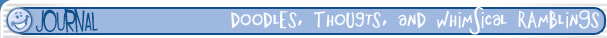## Wednesday, January 12, 2011

### distanceTools.py is kinda workin okay...

Color me surprised. Been workin on this the last two days. Some of the foundation stuff for the project. Still getting used to syntax changes but really enjoying the flexibility python affords.

Also found a better way to display code in posts, woot woot!

``````
"""
distanceTools.py
Josh Burton
www.joshburton.com
1/12/2011

Collection of functions for measuring stuff!

Function Key:
1) distanceBetweenPoints (point1, point2)
return - distance

2) distanceBetweenObjects (obj1, obj2)
return - distance

3) getWSPos (obj)
return - world space position in array format

4) distanceBetweenObjectsArray (objArray)
return - array of distances between each object in the input array

5) averageDistanceBetweenObjects (objArray)
return - average of the distancees between the objects in the input array

6) measureDistanceBetweenObjs (objArray)
creates - measure nodes between all ojects in the list,names them,
checks for a 'measure_grp' and if it finds none, creates it, parents distance nodes under that

"""

""" returns the distance between two points in space """
def distanceBetweenPoints (point1, point2):
from math import sqrt,pow
distance = sqrt( pow(point1-point2, 2) + pow(point1-point2, 2) + pow(point1-point2, 2) )
return distance

""" returns the distance between two object's pivots """
def distanceBetweenObjects (obj1, obj2):
from math import sqrt,pow
point1 = getWSPos (obj1)
point2 = getWSPos (obj2)
distance = sqrt( pow(point1-point2, 2) + pow(point1-point2, 2) + pow(point1-point2, 2) )
return distance

""" simple worldspace position query """
def getWSPos (obj):
pos = cmds.xform (obj,q=True, ws=True, rp=True)
return pos

""" Pass an array of objects into it and get an array of distances """
def distanceBetweenObjectsArray (objArray):
from math import sqrt,pow
cnt = 0
objPositionArray = []
distancesArray = []
cnt = (len(objArray) - 1)
firstTermCnt = 0
seoncTermCnt = 1

for obj in objArray:
"""get its positional data"""
tempPos = getWSPos (obj)
objPositionArray.append (tempPos)

while cnt > 0:
point1 = objPositionArray[firstTermCnt]
point2 = objPositionArray[seoncTermCnt]
distance = sqrt( pow(point1-point2, 2) + pow(point1-point2, 2) + pow(point1-point2, 2) )
distancesArray.append (distance)
firstTermCnt +=1
seoncTermCnt +=1
cnt -= 1

if (cnt == 0):
break

return distancesArray

""" averages the distance between objects in an array"""
def averageDistanceBetweenObjects (objArray):
distanceArray = distanceBetweenObjectsArray (objArray)
average = float(sum(distanceArray)) / len(distanceArray)
return average

""" Makes measure nodes between every object in the array """
def measureDistanceBetweenObjs (objArray):
"""need to add grouping and naming if I wanna use this"""
cnt = 0
locatorPositionArray = []
cnt = (len(locArray) - 1)
firstTermCnt = 0
seoncTermCnt =1
distanceObjArray = []
coreNameArray = []

if objExists ('measure_grp'):
pass

else:
tmp = cmds.group (empty=True, name='measure_grp')
cmds.xform (tmp, os=True, piv= (0,0,0))

for obj in objArray:
"""get its positional data"""
tempPos = cmds.xform (obj,q=True, ws=True, rp=True)
locatorPositionArray.append (tempPos)

tmp = obj.split('_')
coreNameArray.append (tmp)

while cnt > 0:
distanceObj = cmds.distanceDimension( sp=locatorPositionArray[firstTermCnt], ep=locatorPositionArray[seoncTermCnt] )
tmp = cmds.listRelatives ( [distanceObj], p=True)
tmp = cmds.rename (tmp, (coreNameArray[firstTermCnt]+'_to_'+coreNameArray[seoncTermCnt]+'_distMeas') )
distanceObjArray.append (distanceObj)
firstTermCnt +=1
seoncTermCnt +=1
cnt -= 1

cmds.parent (tmp,'measure_grp')

if (cnt == 0):
break

``````

Morgan Loomis said...

Hey dude, just a friendly piece of advice, you should consider putting your notes right after the function definition, rather than before. That way they become docstrings, and show up when you use the help() command.

For example:

def distanceBetweenPoints (point1, point2):
""" returns the distance between two points in space """
from math import sqrt,pow
distance = sqrt( pow(point1-point2, 2) + pow(point1-point2, 2) + pow(point1-point2, 2) )
return distance

#then try running:
help(distanceBetweenPoints)

#and you get:
#distanceBetweenPoints(point1, point2)
# returns the distance between two points in space

And if you ever start generating documentation for your scripts, it will show up there, too. Python's awesome because of stuff like this. Yay!

Unknown said...

Mornin Morgan, thanks for the advice. My buddy had mentioned something about using the """comments""" stuff rather than the #method because of the generating documentation process. However I hadn't figured out how that worked exactly yet. I'll go back and revise the comments in all the stuff I've written so far. Thanks again!
-jjb

All the stuff on this site is 2000 - by Josh Burton...unless otherwise noted. All rights reserved.